### Home > CALC > Chapter 1 > Lesson 1.4.1 > Problem1-141

1-141.
1. Find the domain for each of the following functions. Note: The functions mentioned in parts (c) and (d) refer to those in parts (a) and (b). Homework Help ✎

1.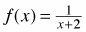2.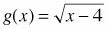3.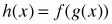4.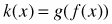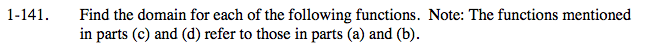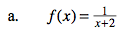$\text{Visualize }f(x).\text{ It is a horizontal shift of }y=\frac{1}{x},$

which has a vertical asymptote at x = 0. f(x) also has a vertical asymptote. Where is it, and how will the vertical asymptote restrict the domain?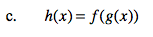$\text{Find the domain of }\left ( \frac{1}{\sqrt{x-4}+2} \right )$

Both the square root and the denominator have restricted domains. Combine.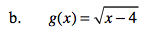$\text{Visualize }g(x).\text{ It is a horizontal shift of }y=\sqrt{x},$

which does not exist for negative values of x. What can you conclude about g(x).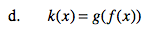Refer to hints in part (c).